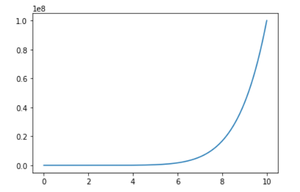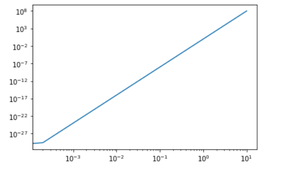# Matplotlib.pyplot.loglog() function in Python

• Last Updated : 06 Jul, 2021

Prerequisites: Matplotlib

Matplotlib is a comprehensive library for creating interactive, static and animated visualizations in python. Using general-purpose GUI toolkits like wxPython, SciPy, Tkinter or SciPy, it provides an object-oriented API for embedding plots into applications. Matplotlib.pyplot is a collection of functions that makes Matplotlib work like MATLAB.

Here, we will be exploring loglog() function of Matplotlib.pyplot. It is used to plot a log scale over both x and y-axis.

Syntax:

`loglog(X,Y)`

Where,

X and Y refer to x and y coordinates respectively.

Other function used is linespace(). It returns evenly spaced numbers over a specified interval.

Syntax:

`np.linspace(start, stop, num, endpoint, retstep, dtype, axis)`

Where,

• Start : The starting value of sequence from where you want to show the line, or we can say starting point of line
• Stop : It is the end value of the sequence at where the line stops, unless ‘endpoint’ is set to False.
• Num : Number of samples to generate. Must be non-negative. By default, it is 50.
• Endpoint : It works same as stop. If it is True then stop is the last sample else stop is excluded from the sequence.
• Retstep : If True, return (‘samples’, ‘step’), where `step` is the spacing between samples.
• Dtype : The type of the output array.
• Axis : The axis in the result to store the samples and it is relevant only if start or stop are array-like

Example : Without loglog()

## Python

 `# importing required modules``import` `matplotlib.pyplot as plt``import` `numpy as np`  `# inputs to plot using loglog plot``x_input ``=` `np.linspace(``0``, ``10``, ``50000``)` `y_input ``=` `x_input``*``*``8` `# plotting the value of x_input and y_input using plot function``plt.plot(x_input, y_input)`

Output:Example : With loglog()

## Python3

 `# importing required modules``import` `matplotlib.pyplot as plt``import` `numpy as np`  `# inputs to plot using loglog plot``x_input ``=` `np.linspace(``0``, ``10``, ``50000``)` `y_input ``=` `x_input``*``*``8` `# plotting the value of x_input and y_input using loglog plot``plt.loglog(x_input, y_input)`

Output:My Personal Notes arrow_drop_up